# Oblique asymptotes online calculatorThe straight line y = k x + b is the oblique asymptote of the function f(x), if the following condition is hold: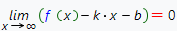On the basis of the condition given above, one can determine the coefficients k and b of the oblique asymptote of the function f(x):<=>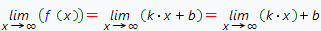then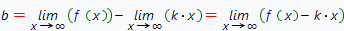and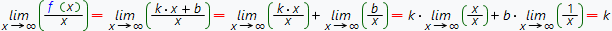Thus the straight line y = k x + b is the oblique asymptote of the function f(x) if and only if the finity limits exist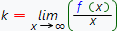and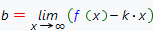To find oblique asymptotes of your function, you can use our free online calculator, based on the Wolfram Alpha system.

Oblique asymptotes calculator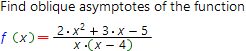Function which oblique asymptotes you want to find:

Install calculator on your site

## Other useful links:

Advanced math input panel working rules
Limits calculation formulas